Courses

# Test: Heterocyclic Level - 1

## 20 Questions MCQ Test Organic Chemistry | Test: Heterocyclic Level - 1

Description
This mock test of Test: Heterocyclic Level - 1 for Chemistry helps you for every Chemistry entrance exam. This contains 20 Multiple Choice Questions for Chemistry Test: Heterocyclic Level - 1 (mcq) to study with solutions a complete question bank. The solved questions answers in this Test: Heterocyclic Level - 1 quiz give you a good mix of easy questions and tough questions. Chemistry students definitely take this Test: Heterocyclic Level - 1 exercise for a better result in the exam. You can find other Test: Heterocyclic Level - 1 extra questions, long questions & short questions for Chemistry on EduRev as well by searching above.
QUESTION: 1

### Pyrrole is less basic than pyridine. The orbital having the lone pair of electrons on nitrogen in pyrrole is:

Solution:
• The nitrogen atom is sp2  hybridized. The N electron pair is on a p orbital, perpendicular to the plane of the ring and overlapping with the other p orbitals.
• There are a total of 6 π electrons, which is a Hückel number (Hückel numbers are 2, 6, 10, etc.) and, therefore, the molecule is aromatic.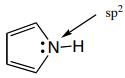QUESTION: 2

Solution:
QUESTION: 3

### Furan on prolonged heating with dimethyl acetylenedicarboxylate yields:

Solution:
QUESTION: 4

The structures of the products A and B formed in the above reaction scheme respectively are: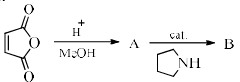Solution:
QUESTION: 5

The decreasing order of the reactivity of the following compounds towards electrophiles is: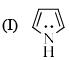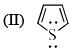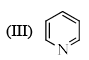Solution:

Pyrole is most reactive towards the electrophiles because in this case it is carrying one lone pair of electron .and it is attached with one hydrogen atom , resulting less resonance stabilize than that of pyridine one the another hand thiophene is more reactive because it's carry 2 lone pair of electrons at last pyrole is more reactive than thiophene because nitrogen is smaller in size than that of sulphur so the availability of electrons cloud is maximum than sulphur ... consequently it is highly reactive towards electrophiles

QUESTION: 6

Pyridine undergoes electrophilic nitration at elevated temperature to given the following as a major product:

Solution: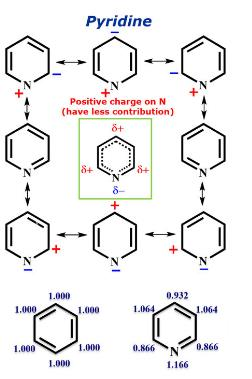There is a relatively less partial positive charge on meta positions of Pyridine. Hence, when an electrophile attacks it attacks on meta position.
Hence D is correct.

QUESTION: 7

A pyridine derivative (P) reacts with (Y). (Y) can be a free radical, cation or anion. The structure of intermediate (Q) formed in the reacts is given below. (P) and (Y) respectively are: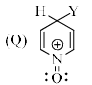Solution:
QUESTION: 8

Pyrrole + PhMgBr → E + F
E + MeCl → G + H
F + MeCl → no reaction without a catalyst.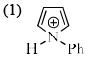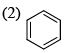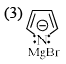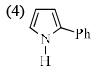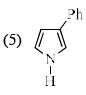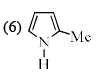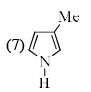The structure of products E–H, respectively are:

Solution:
QUESTION: 9

Chose the correct answer from the following four choices.
Statement: Pyridine is more basic than pyrrole.
Reason: The nitrogen in pyrrole carries a proton while the nitrogen in pyridine does not.
Assertion: Nitrogen in trigonal geometry are generally more basic than nitrogens in a tetrahedral geometry.

Solution:
• Pyridine is more basic than pyrrole because lone pair of electrons on N in pyridine and pyrrole are different in nature. These form a part of aromatic sextet in pyrrole, while not in pyridine.
• Pyrrole, C4H4NH (in which N contributes a lone pair) has a pKa−3.8 but pyridine (where N is part of the ring's double bond) has a pKa 5.14. Electron pair availability indicates the strength of basicity. In this case, pyridine is the stronger base.
• In pyrrole, the lone pair electrons of the nitrogen atom gets involved with the 2 carbon-carbon double bonds in the 5-member ring to form a conjugated system of pi electrons, leading to greater stability of the molecule.
• Pyridine, on the other hand, already has a stable conjugated system of 3 double bonds in the aromatic hexagonal ring, like benzene. Hence, the lone pair electrons on the N atom in pyridine can be easily donated to a H+ ion or a Lewis acid.
QUESTION: 10

In the following sequence of reactions, the major product Q is: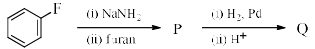Solution:
QUESTION: 11

What will be the reagent used for the completion of the following reaction?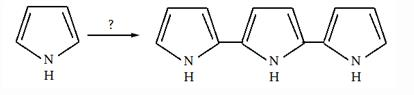Solution:
QUESTION: 12

The correct order of the basicity of the following compound is: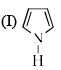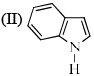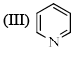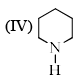Solution:
QUESTION: 13

Identify the major Product P in the following two–step reaction: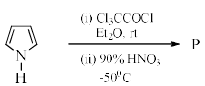Solution:
QUESTION: 14

Match the structures in List–I with their correct names in List–II.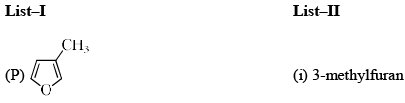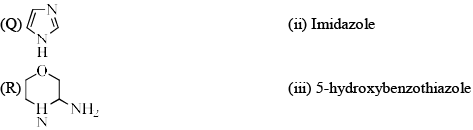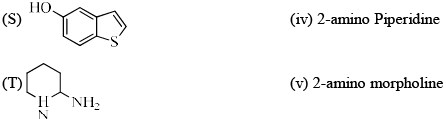Solution:
QUESTION: 15

The most acidic species is:

Solution:
QUESTION: 16

The compound that is NOT oxidized by KMnO4 is:

Solution:
QUESTION: 17

Thiophene reacts with HCHO in presence of aqueous HCl to give:

Solution:

The CH2O/HCl reagent is suitable for chloromethylation of various polycyclic aromatic hydrocarbons as well as heterocyclic aromatic compounds. Thiophene gives 2-(chloromethyl)thiophene in 40-41% isolated yield, and 4-methylimidazole gives the 5-chloromethyl derivative (51-68%).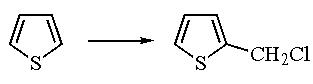QUESTION: 18

The reaction of 2-methylfuran with DMF-POCl3 would give:

Solution:
QUESTION: 19

In the following reaction the major product (X) is: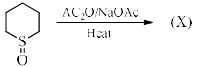Solution:
QUESTION: 20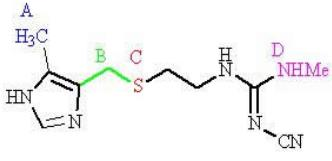Two regions of cimetidine are susceptible to metabolism. Which regions?

Solution: